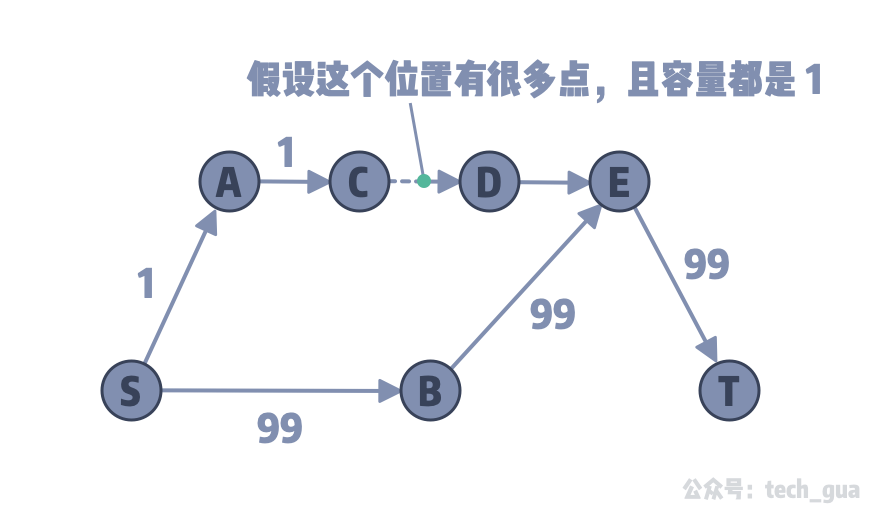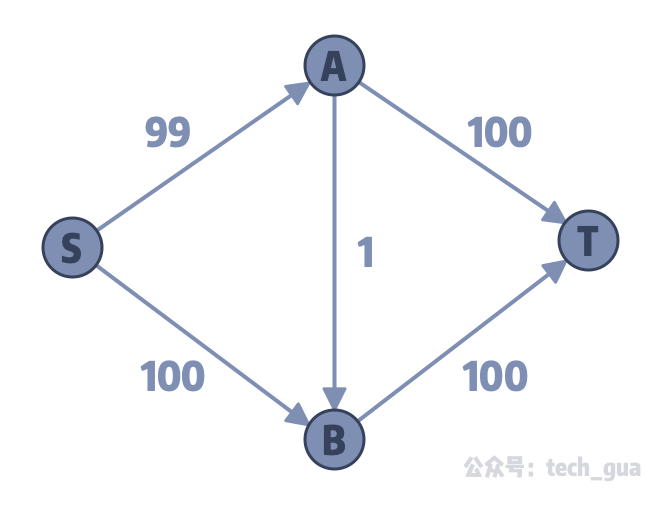# 知识梳理

• 在「初识最大流问题」中，我们了解了什么是流网络模型、什么是最大流问题、以及在流网络中 的增广路（Augmenting Path）概念
• 在「Ford-Fulkerson 最大流求解方法」中，我们学习了 Ford-Fulkerson 的最大流问题求解方法和思路：不断的深度优先搜索，直到没有增广路为止则获得最大流
• 在「二分匹配的最大流思维」中，通过增加超级源和超级汇来修改二分图，从而将二分匹配问题转换成了最大流问题，最后通过 Ford-Fulkerson 方法解决。

# Ford-Fulkerson 方法有什么问题？

1. 使用 DFS 搜索 出一条增广路；
2. 在这条路径中所有的边的容量减去这条增广路的流量 f，并建立容量为 f 的反向边；
3. 返回操作一，直到没有增广路；# 算法导论上给出的最坏情况分析#include <iostream>
#include <vector>
#include <queue>
#include <unordered_map>
using namespace std;

#define INF 0x3f3f3f3f

const int MAX_V = 1e4 + 5;

struct Edge {
int to, cap, rev;
Edge(int _to, int _cap, int _rev): to(_to), cap(_cap), rev(_rev) {}
};

// 邻接表记录点和其相连的边
// 比如节点 1 的所有出度边集 G
vector<Edge> G[MAX_V];

void add_edge(int from, int to, int cap) {
G[from].push_back(Edge(to, cap, G[to].size()));
G[to].push_back(Edge(from, 0, G[from].size() - 1));
}

bool used[MAX_V];

int dfs(int c, int t, int f) {
if (c == t) {
return f;
}
used[c] = true;
for (int i = 0; i < G[c].size(); ++ i) {
Edge &e = G[c][i];
if (!used[e.to] && e.cap > 0) {
int d = dfs(e.to, t, min(f, e.cap));
if (d > 0) {
e.cap -= d;
G[e.to][e.rev].cap += d;
return d;
}
}
}
return 0;
}

int max_flow(int s, int t) {
int flow = 0;
int cnt = 0;
for (;;) {
memset(used, 0, sizeof(used));
int f = dfs(s, t, INF);
cnt += 1;
if (f == 0) {
cout << cnt << endl; // 5 - 也就是说只进行了 5 次增广路查询
return flow;
}
flow += f;
}
}

int main() {
// 0: S点
// 1: A点
// 2: B点
// 3: T点
int ret = max_flow(0, 3);

cout << ret << endl; // 199
}


int d = dfs(e.to, t, min(f, e.cap));


# 基于 DFS 的 FF 方法复杂度分析

$O(V·F)$

# Edmond-Karp 算法解析1. 使用 BFS 找到一条增广路（对应下面的步骤 1）；
2. 计算这条路的最小容量边，为汇点加流量，并建立反向边，其容量为增加的流量（对应下面的步骤 2）；
3. 重复第一步，如果不能找到一条增广路则得到最大流；

## 1. BFS 搜索查询增广路// 用来记录当前路径上的最小容量，用于加流量操作
int a[MAX_V];

// 记录下标点的边编号，pair 对应 G[x1][x2]，x1 是描述哪个入度点，x2 是描述 x1 点的第 x2 条边
unordered_map<int, pair<int, int>> pre;


void bfs(int s, int t) {
// a 初始化成 0，也可以判断是否已经被染色，从而剪枝情况
memset(a, 0, sizeof(a));
// 使用队列，保存处理节点
queue<int> que;
que.push(s);
// 每个节点所流过的流量设置为 INF 无穷大
// 这样可以起到求最小的作用
a[s] = INF;
while (!que.empty()) {
int x = que.front();
que.pop();
// 遍历当前节点的所有边
for (int i = 0; i < G[x].size(); ++ i) {
Edge& e = G[x][i];
// 如果相连的点没有访问，并且这条边的容量大于 0
if (!a[e.to] && e.cap > 0) {
// 记录下一个点的入度边
pre[e.to] = make_pair(x, i);
// 计算当前路径的最小容量
a[e.to] = min(a[x], e.cap);
que.push(e.to);
}
}
if (a[t]) break;
}
}


## 2. 增广路径上的边与反向边的容量操作int max_flow(int s, int t) {
// 最大流结果
int ret = 0;
while (1) {
// 从 S -> T 使用 bfs 查询一条增广路
bfs(s, t);
// 如果发现容量最小是 0 ，说明查不到了
if (a[t] == 0) break;
int u = t;
while (u != s) {
// 使用 pre 来获取当前增广路中汇点 T 的入度边下标信息
int p = pre[u].first, edge_index = pre[u].second;
// 获取正向边和反向边
Edge& forward_edge = G[p][edge_index];
Edge& reverse_edge = G[forward_edge.to][forward_edge.rev];
// 更新流量
forward_edge.cap -= a[t];
reverse_edge.cap += a[t];
// 逆增广路方向移动游标继续更新
u = reverse_edge.to;
}
ret += a[t];
}
return ret;
}


# EK 最大流完整实现#include <iostream>
#include <vector>
#include <queue>
#include <unordered_map>
using namespace std;

#define INF 0x3f3f3f3f

const int MAX_V = 1e4 + 5;

struct Edge {
int to, cap, rev;
Edge(int _to, int _cap, int _rev): to(_to), cap(_cap), rev(_rev) {}
};

vector<Edge> G[MAX_V];
int a[MAX_V];
unordered_map<int, pair<int, int>> pre;

void add_edge(int from, int to, int cap) {
G[from].push_back(Edge(to, cap, G[to].size()));
G[to].push_back(Edge(from, 0, G[from].size() - 1));
}

void bfs(int s, int t) {
memset(a, 0, sizeof(a));
queue<int> que;
que.push(s);
a[s] = INF;
while (!que.empty()) {
int x = que.front();
que.pop();
for (int i = 0; i < G[x].size(); ++ i) {
Edge& e = G[x][i];
if (!a[e.to] && e.cap > 0) {
pre[e.to] = make_pair(x, i);
a[e.to] = min(a[x], e.cap);
que.push(e.to);
}
}
if (a[t]) break;
}
}

int max_flow(int s, int t) {
int ret = 0;
while (1) {
bfs(s, t);
if (a[t] == 0) break;
int u = t;
while (u != s) {
int p = pre[u].first, edge_index = pre[u].second;
Edge& forward_edge = G[p][edge_index];
Edge& reverse_edge = G[forward_edge.to][forward_edge.rev];
forward_edge.cap -= a[t];
reverse_edge.cap += a[t];
u = reverse_edge.to;
}
ret += a[t];
}
return ret;
}

int main() {
int ret = max_flow(0, 5); // 输出 23
cout << ret << endl;
}



# EK 算法时间复杂度及适用情况

$O(V·E^2)$

$T_{FF} = O(E·F)$ $T_{EK}=O(V·E^2)$

1. 当流网络中边少的时候，或者说是一个稀疏图（E < VlogV）时，我们可以选用 EK 算法进行最大流求解；
2. 当流网络中边多的时候，或者说是一个稠密图（E > VlogV）时，可以选用 FF 方法求解。

# 总结